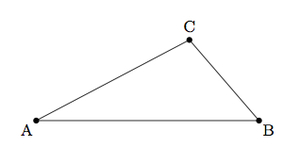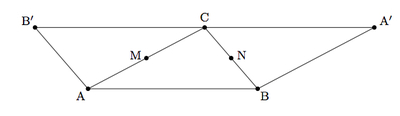# Sum of angles in a triangle

Alignments to Content Standards: G-CO.C.10

Suppose $ABC$ is a triangle in the plane as pictured below:Suppose $M$ is the midpoint of $\overline{AC}$ and $N$ is the midpoint of $\overline{BC}$.

1. Draw the rotation of $\triangle ABC$ by 180 degrees about $M$, labeling the image of $B$ as $B^\prime$.
2. Draw the rotation of $\triangle ABC$ by 180 degrees about $N$, labeling the image of $A$ as $A^{\prime}$.
3. Explain why $B^\prime$, $C$, and $A^{\prime}$ are collinear.
4. Deduce that $m(\angle A) + m(\angle B) + m(\angle C) = 180$.

## IM Commentary

The goal of this task is to provide an argument, appropriate for high school students, for why the sum of the angles in a triangle is 180 degrees. Students see this result in the eighth grade (https://www.illustrativemathematics.org/illustrations/1501) where it is presented in a less formal, grade appropriate fashion. Here students use the formal properties of rotations (namely that they preserve angle measures) to deduce that the sum of the angles in the triangle is 180 degrees. The parallel postulate (that given a line $\ell$ and a point $P$ not on $\ell$, there is one and only line parallel to $\ell$ and containing $P$), and the properties of rigid motions are used in part (c).

The approach taken here is more demanding as only the given triangle is pictured and the rest of the construction is done dynamically from there. The teacher can also give students the parallel line to $\overleftrightarrow{AB}$ through $C$ (as in the eighth grade task) and then have students carefully reason from there to arrive at the conclusion in part (d).

## Solution

1. Below is a picture showing the midpoints of $\overline{AC}$ and $\overline{BC}$ as well as the rotated images of $\triangle ABC$, by 180 degrees about $M$ and $N$:Since the rotation about $M$ is a 180 degree rotation, it maps $\overleftrightarrow{AC}$ to itself. Since $M$ is the midpoint of $\overline{AC}$ it maps $A$ to $C$ and $C$ to $A$. The point $B$ maps to $B^\prime$ which has the property that $M$ is the midpoint of $\overline{BB^\prime}$.

2. This is also pictured above. As in the part (a), this rotation interchanges $B$ and $C$ and maps $A$ to the point marked $A^\prime$.
3. We know that 180 degree rotations about $M$ and $N$ map  $\overleftrightarrow{AB}$ to lines which are parallel to $\overleftrightarrow{AB}$. The rotation about $M$ maps $\overleftrightarrow{AB}$ to $\overleftrightarrow{CB^\prime }$ while the rotation about $N$ maps $\overleftrightarrow{AB}$ to $\overleftrightarrow{CA^\prime }$. Both of these lines are parallel to $\overleftrightarrow{AB}$ and contain $C$. The parallel postulate establishes that $\overleftrightarrow{CB^\prime} = \overleftrightarrow{CA^\prime}$. This means that $A^\prime$, $B^\prime$, and $C$ are collinear.
4. Rotations preserve angle measures so $m(\angle B^\prime CA) = m(\angle BAC)$ and $m(\angle BCA^\prime) = m(\angle CBA)$. We have $$m(\angle B^\prime CA) + m(\angle ACB) + m(\angle BCA^\prime) = 180$$ since these three angles make a line. Substituting the equivalent angles gives $$m(\angle BAC) + m(\angle ACB) + m(\angle CBA) = 180$$ as desired.